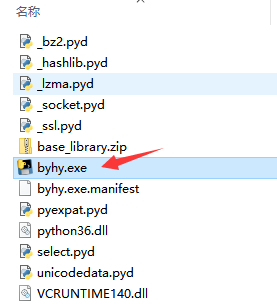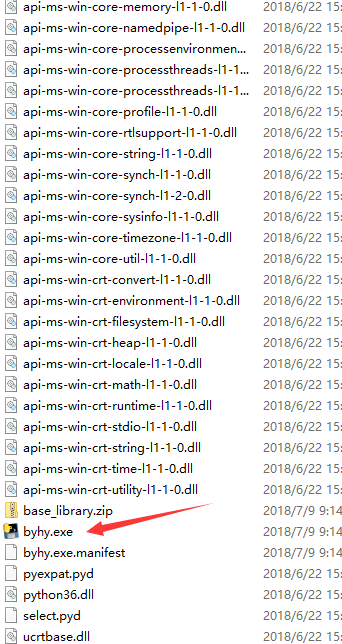# 制作成可执行文件

1. 安装Python解释器
2. 在命令行运行python程序文件，比如： python xxx.py

PyInstaller 支持 Python 2.7 和 Python 3.3 以后的版本。

## 命令行程序打包

``````pip install pyinstaller
``````

``````welcome = '''

########################################
#                                      #
#     白月黑羽 PyInstaller 演示程序    #
#                                      #
########################################

'''

print(welcome)

while True:
exp = input('\n\n请输入一个数学运算式 [输入quit退出]：')
if exp == 'quit':
break
try:
result = eval(exp)
except:
print('\n！！无效的运算式')
continue

print(f'结果为: {result}')
``````

``````pyinstaller byhy.py --workpath d:\pybuild  --distpath d:\pybuild\dist
``````## 将库文件单独存放在目录中

``````import sys
import os

currentdir = os.path.dirname(sys.argv)
libdir = os.path.join(currentdir, "lib")
print(currentdir)
sys.path.append(libdir)
os.environ['path'] += ';./lib'
``````

``````pyinstaller byhy.py --workpath d:\pybuild  --distpath d:\pybuild\dist --runtime-hook="runtimehook1.py"
``````

• Python库搜索路径，这是由 `sys.path.append(libdir)` 指定的。

• 动态链接库文件的 搜索路径，这是由 `os.environ['path'] += ';./lib'` 指定的。

``````base_library.zip
byhy.exe
byhy.exe.manifest
python36.dll
``````

## 图形界面程序打包

``````pyinstaller guitool.py --noconsole --workpath d:\pybuild  --distpath d:\pybuild\dist
``````